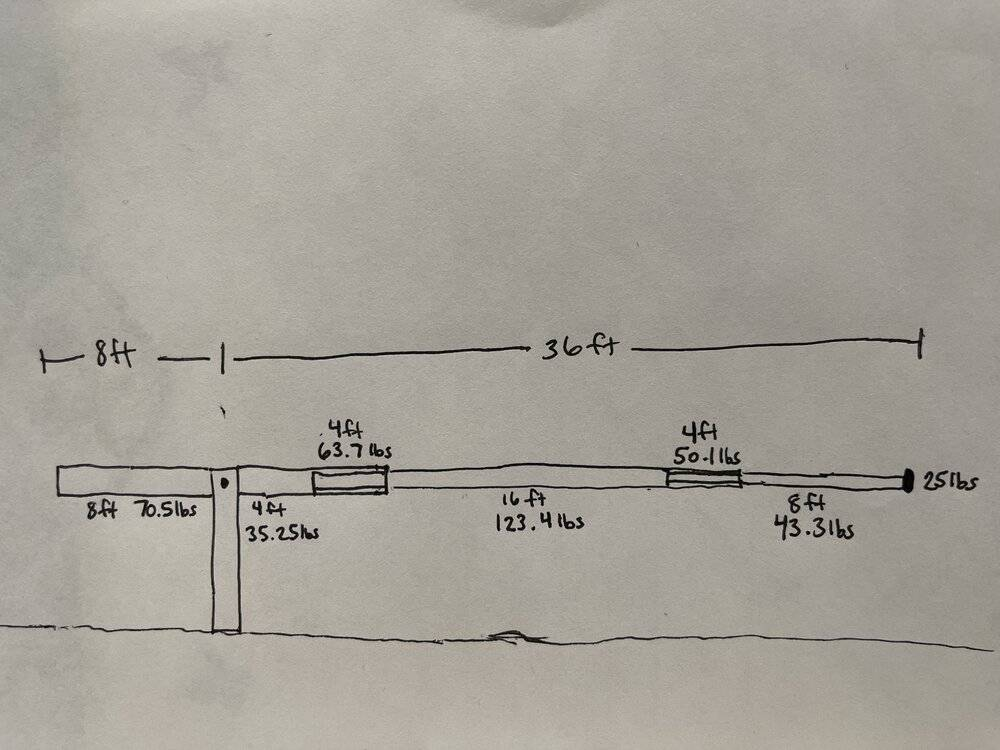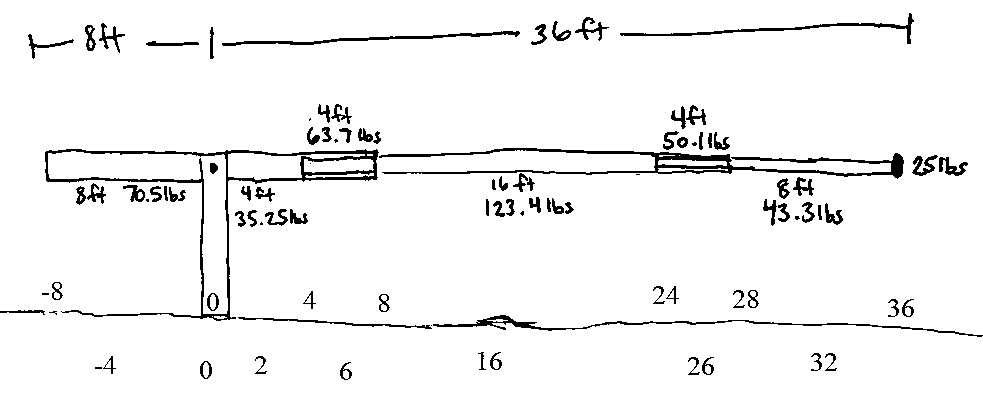# How much counterweight?

kjmdrumz3
TL;DR Summary
I’m roughing out a design for a self-supporting antenna mast and need someone smarter than me to help me with the counterweight. Assuming an 8ft pivot point, a 25lb load on the top of the long end and given dimensions and weights, how much counterweight is needed to balance? Thanks!Mentor
Welcome to PhysicsForums.To balance the "teeter-totter", you need to have the torques for the two sides cancel out.

Each torque is the force (weight) multiplied by the distance from the pivot point. If you have a 10 pound weight that is 10 feet out from the pivot point, you would need a 20 pound counterweight at 5 feet out the other way to balance it.

So in your drawing, add up the torques on the right side from each section's weight (at the center of mass for that weight), and divide that total by the 8' distance on the left to tell you what counterweight needs to be put there to balance the system.

Also, be sure that whatever locking mechanism you put at the base of the antenna mast support is very solid and sure. Don't ask me why I'm pointing this out (it has to do with a very large antenna a few years ago at Field Day...).

kjmdrumz3
So, by my basic math, I’m getting a counterweight of almost 700-800 pounds. Does that seem correct?

Mentor
I'm having a little trouble understanding your diagram so far. For me to help check your numbers, could you add a dot at the center of mass of each section on the right, and label it with the weight of that section? If you could also add the distance out to each CoM dot from the pivot, that would help. Thanks.

Is this a HAM antenna BTW?Also is there a possibility of lifting the mast using a rope to the top that goes through a pulley on something to the left (like a tree or a building)?

Mentor
Also, is that 70.5# for the section to the left of the pivot? Its CoM would be 4' to the left of the pivot, correct?

Staff Emeritus
I’m getting a counterweight of almost 700-800 pounds. Does that seem correct?

Why does that surprise you? Your item weighs over 400 pounds.

Gold Member
There's a key concept that @berkeman referred to: You can calculate the torque of a section by finding the center of mass times the distance to that center of mass. The center of mass of a uniform beam section is the mass of that section located at the middle of the section. You can also add the torques from each section to get the total.

So, if you have a cantilevered beam that is 20kg from 0-1m and 10kg from 1-3m, then the total torque would be = 20kg⋅(1m - 0m)/2 + 10kg⋅(3m - 1m)/2 = 10kg-m + 10kg-m = 20kg-m. 20kg⋅(1m + 0m)/2 + 10kg⋅(3m + 1m)/2 = 10kg-m + 20kg-m = 30kg-m. Then you will want to balance this with the same torque on the other side which could be either a 10kg 15kg weight at 2m, or a 20kg 30kg beam 2m long.

edit: oops! typo fixed. Good thing I don't have to take physics exams anymore!

Last edited:
1'st, mark the x values along the tower.
2'nd, mark the centres of mass. Here is the diagram with CofM marked.3'rd, sum the products of mass and CofM, negative to the left, don't forget the top load.
(-4*70.5)+(2*35.25)+(6*63.7)+(16*123.4)+(26*50.1)+(32*43.3)+(36*25) = 5733.3 ft lbs

To balance, that requires a mass at -4 ft of 5733.3/4 = 1433. lbs

Gold Member
To balance, that requires a mass at -4 ft of 5733.3/4 = 1433. lbs
So, a 1959 Austin mini (including driver) hanging off the left of that antenna. Seems feasible.

So, a 1959 Austin mini - plus driver - hanging off the back end. Seems feasible.
That is the answer to the question "How can a 1959 Austin mini develop a torque of 5733 ft lbs." ?

•Mentor
To balance, that requires a mass at -4 ft of 5733.3/4 = 1433. lbs
No, cut the Mini in half and hang it on the end 8' out...That is the answer to the question "How can a 1959 Austin mini develop a torque of 5733 ft lbs." ?

I’m getting a counterweight of almost 700-800 pounds. Does that seem correct?
No. You will need close to twice that because it cannot all be at the foot of the tower.
You might consider making a steel box for ballast that hangs from a pin, say at the –4 ft mark. That keeps the box off the ground when the tower is erect. Drill some drain holes in the corners and cover it with a lid. The box can then be filled with steel ballast until the tower begins to rise. Ballast is less likely to fall off when in a swung box. Ballast can be adjusted to counteract later changes in the load. How big will that box need to be?
1433 lb / 2.2046 = 650 kg. The density of iron is 7.8; So one litre of iron weighs 7.8 kg.
It will require about 83.3 litre of solid iron hanging at the –4 ft mark.
Make the box about 110 litre to allow for gaps when packing the scrap.
If you can make a lower profile box, and move it closer to the foot, you can reduce the mass and volume.

kjmdrumz3
Thank you for all of the replies! Looks like a slight redesign using lighter material, or forgetting the counterweight and using a crank is in order. I appreciate all the help!

•berkeman
Gold Member
Oh, and remember the pivot needs to support the total tower weight plus the ballast.

•kjmdrumz3
Oh, and remember the pivot needs to support the total tower weight plus the ballast.
The wind forces on antenna towers are usually greater than the weight of the tower. The vector sum of the tower weight and the wind force must be handled by the pivot pin, while the locking pin handles the wind force only. Those two pins should be as far apart as can be arranged.

Gold Member
My sailing cruiser had a 10m mast that was slightly tapered and, weight about 70kg (bathroom scales at each end). I used to raise and lower it with an A frame that was nearly 2m radius and used the forestay, attached to an eye at deck level. It was straightforward for two men to do it. The A frame gave a considerable mechanical advantage but was necessary because the pivot was at deck level. Even with your 'tabernacle' arrangement, an A frame could still reduce the amount of counterweight needed. There are many images like this one and you could avoid the need for a nearby "tree". Google Mast Lowering with A frame for more ideas.

That must be a pretty beefy tabernacle if all that stuff is up in the air with no guys.•berkeman
Staff Emeritus
an A frame could still reduce the amount of counterweight needed
Excellent advice. Much simpler and more effective than all the earlier posts.

•sophiecentaur